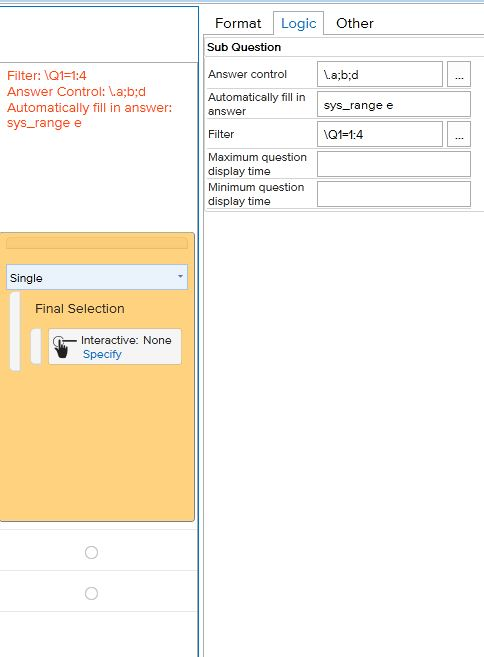# Leastfill

For example you want to show two concepts depended on answers from Q1.

IF Q1 = only answer 1 show Concept1

IF Q1 = only answer 2 show Concept2

IF Q1 = 1,2,3 or 4 show Concept1 or Concept2 depended on the Lowest Quota

IF Q1 = only 3 or 4 show Concept1 or Concept2 depended on the Lowest Quota

You can set this up by creating subquestions

We create one main question called QTLeastFillSelect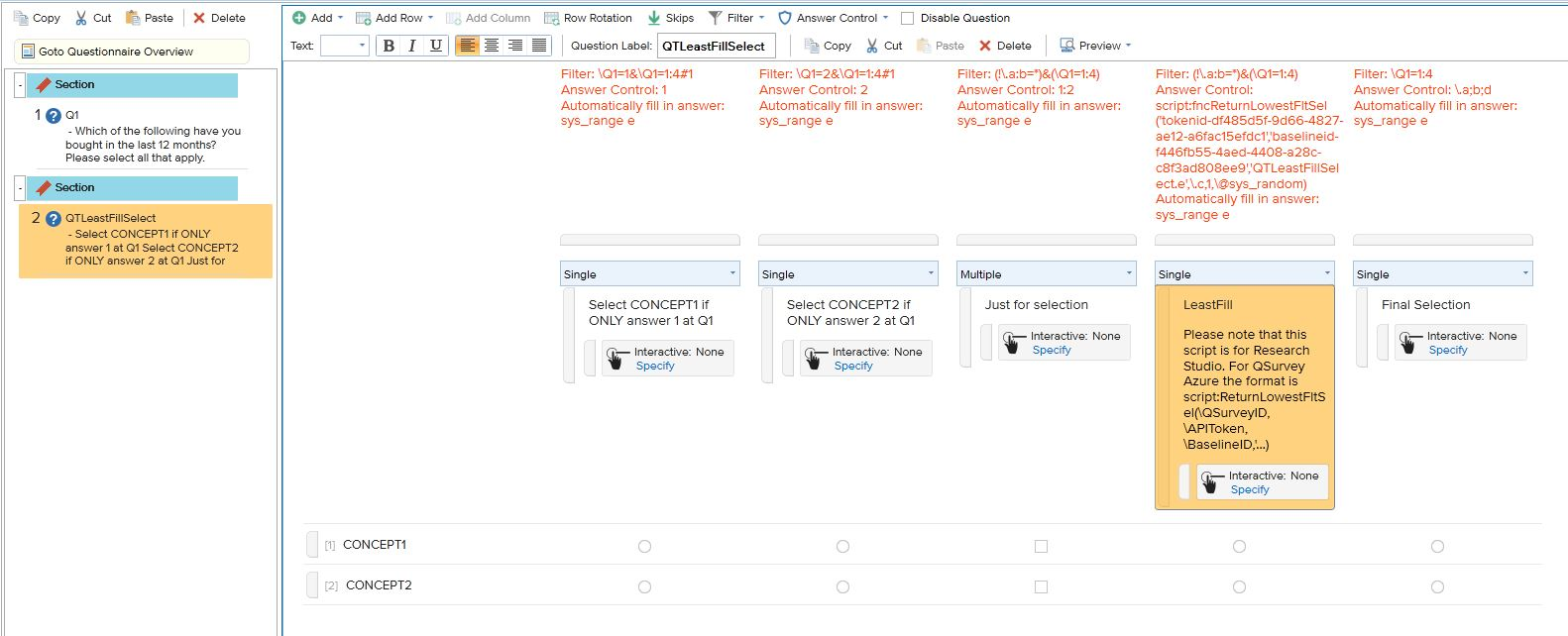The first subquestion (a) is:

Filter: \Q1=1 (answer 1 from Q1) & (AND) Q1=1:4#1 (Only 1 answer)

sys_range e (show question - change to sys_range c to hide)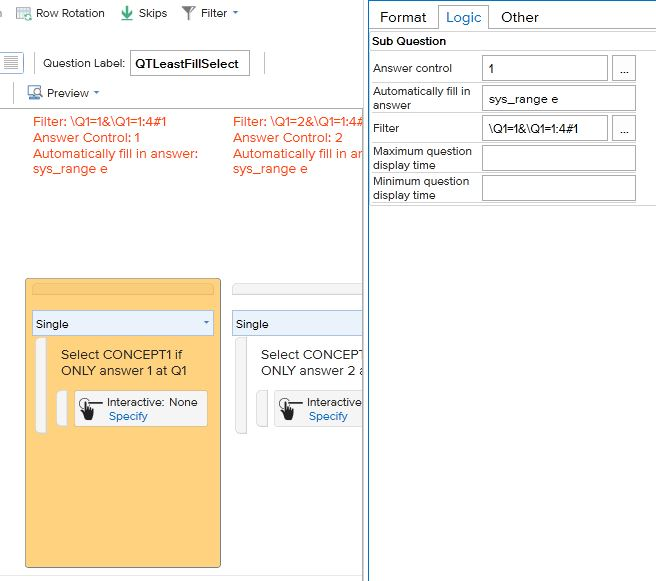The second subquestion (b) is:

Filter: \Q1=2 (answer 2 from Q1) & (AND) Q1=1:4#1 (Only 1 answer)

sys_range e (show question - change to sys_range c to hide)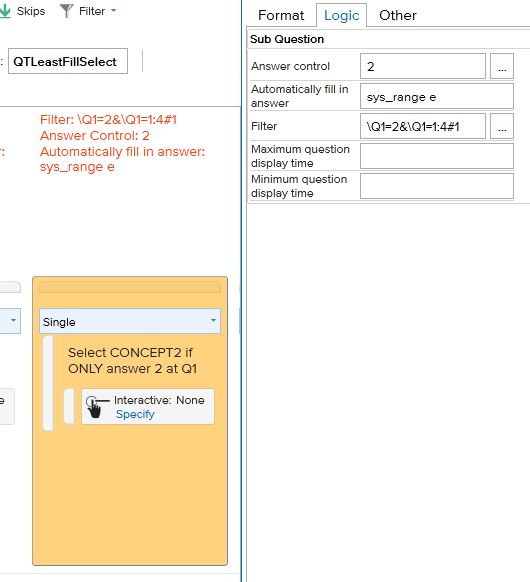The third subquestion (c) is:

Filter: (!\.a:b=*) means NOT an answer at subquestion a or b

& (AND)

(\Q1=1:4) means any answer 1,2,3,4 at Q1

sys_range e (show question - change to sys_range c to hide)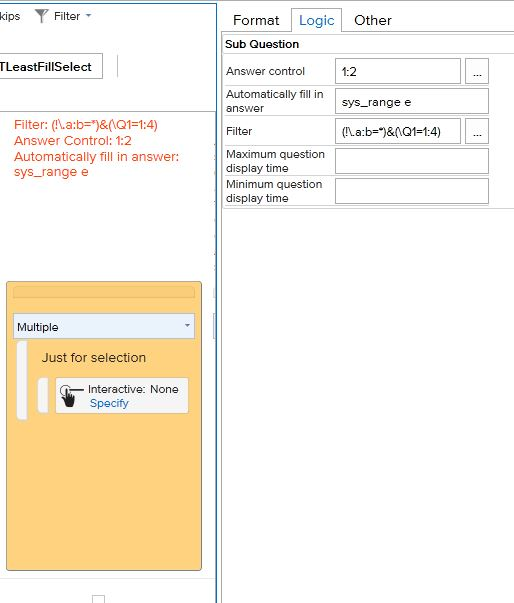The fourth subquestion (d) is:

Filter: (!\.a:b=*) means NOT an answer at subquestion a or b

Answer Control: script is used to get the lowest value of subquestion c and autopunch this in subquestion e

script:ReturnLowestFltSel(\QSurveyID,\APIToken,\BaselineID,'QTLeastFillSelect.e',\.c,1,\@sys_random)

To determine the values for QSurveyID, APIToken and BaselineID:

APITOKEN: (you can request this from QuenchTec - if you have admin access you can see this for any user created in QSurvey (https://login.quenchtec.net/)

BASELINEID: click on the details of your project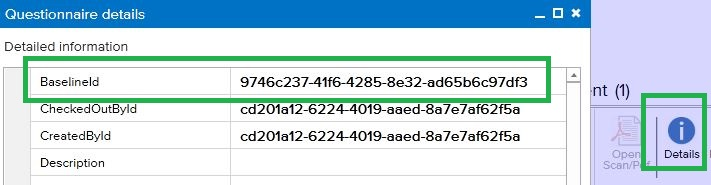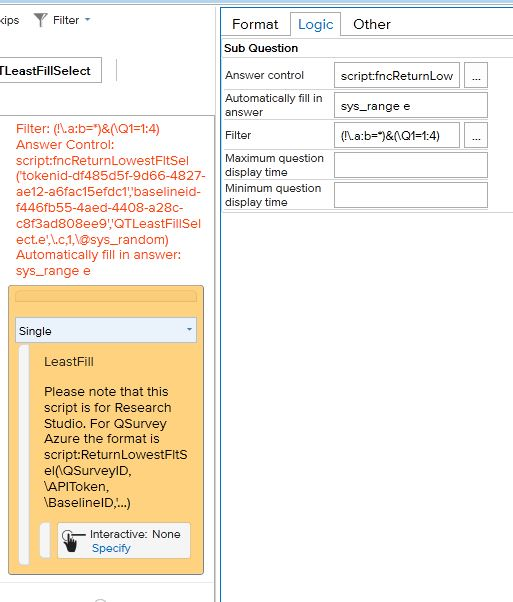The fifth subquestion (e) is:

Autopunch answer from subquestion a, b or d

Filter if Q1 is 1,2,3 or 4